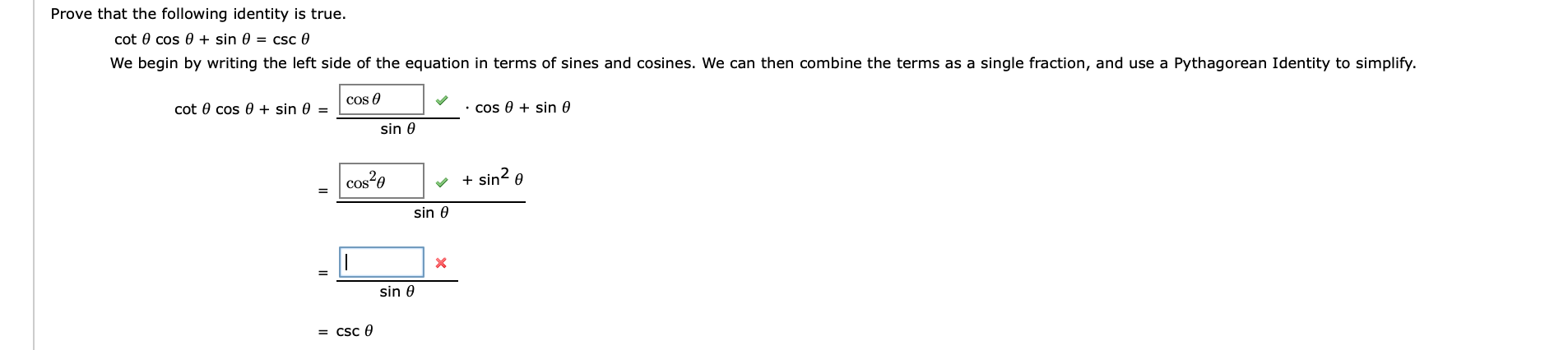# Prove that the following identity is true.cot 0 cos 0 + sin 0 = csc 0We begin by writing the left side of the equation in terms of sines and cosines. We can then combine the terms as a single fraction, and use a Pythagorean Identity to simplify.cot 0 cos 0 + sinCOs sin e=sin 0+ sin 0cos20sin 0Xsin 0= CSC 0

Question
18 viewshelp_outlineImage TranscriptioncloseProve that the following identity is true. cot 0 cos 0 + sin 0 = csc 0 We begin by writing the left side of the equation in terms of sines and cosines. We can then combine the terms as a single fraction, and use a Pythagorean Identity to simplify. cot 0 cos 0 + sin COs sin e = sin 0 + sin 0 cos20 sin 0 X sin 0 = CSC 0 fullscreen
check_circle

Step 1

The given ident...

### Want to see the full answer?

See Solution

#### Want to see this answer and more?

Solutions are written by subject experts who are available 24/7. Questions are typically answered within 1 hour.*

See Solution
*Response times may vary by subject and question.
Tagged in

### Other